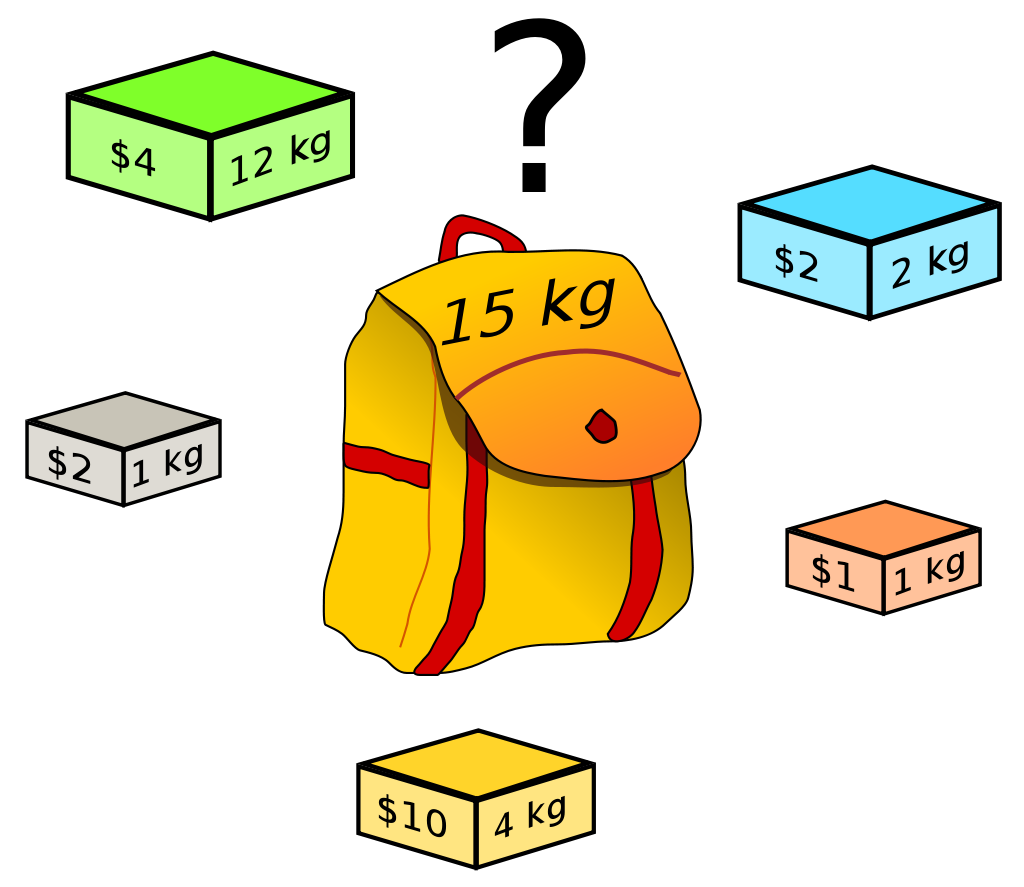# Dynamic Programming::Knapsack Problem

Knapsack problem is a combinatorial optimization problem in Dynamic Programming.

The wikipedia link above explain the formal definition of Knapsack ProblemAnd, based on the illustration of the problem statement, I’ve implemented the knapsack problem in java. Before diving into the implementation, please read the problem definition to solve by yourself.

``````public class KnapsackProblem {

class Items{
public int weight;
public int value;

public Items(int weight, int value) {
this.weight = weight;
this.value = value;
}
}

public static void main(String[] args) {

KnapsackProblem knapsackProblem = new KnapsackProblem();
knapsackProblem.solution();
}

public void solution(){
Items item1 = new Items(1, 6);
Items item2 = new Items(2, 10);
Items item3 = new Items(3, 12);
Items[] items = new Items;
items = item1; items = item2; items = item3;

int maxW = 5;
System.out.println(knapsnack(items, maxW));
}

private int[][] knapsnack(Items[] items, int maxW) {
int[][] cache = new int[items.length + 1][maxW + 1];

for (int i=1; i<items.length; i++){
for (int j=0; j<maxW; j++){
if (items[i-1].weight > j)
cache[i][j] = cache[i-1][j];
else {
cache[i][j] = Math.max(cache[i-1][j], cache[i-1][j-items[i-1].weight] + items[i-1].value);
}
}
}
return cache;
}
}
``````

Couldn’t share the Github link due to NDA with partners associated with LeetCode and HackerRank.

Tags:

Updated: